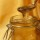Wall Posting18 days
P r o g r e s s s a v e d > -E x i t g a m e -R e t u r n t o c h e c k p o i n t -R e s t a r t E x i t i n g g a m e ~ c a r a m e l h a s l e f t t h e g a m e18 days
G a m e o v e r commented on this post.
":)) I think im gonna save the game, and take a break to cool down. "18 days
L i f e i s l i k e a g a m e, e v e r y g a m e h a s t o c o m e t o a n e n d. G a m e o v e r